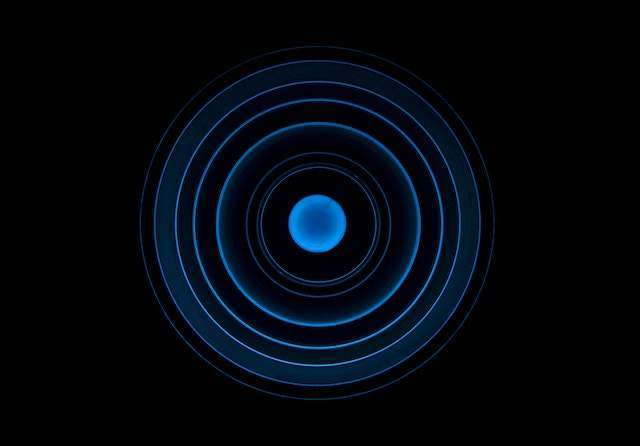# Radius of a Circle Calculator

Instructions: Use this calculator to compute the radius of a circle from its circumference or area, showing all the steps. Please type in value, and indicate whether it is its perimeter or its area that is given, in the form below.Enter the value of the circumference or area (Ex: 3*pi, etc.)

The value provided is the known circumference
The value provided is the known area

This calculator will allow you to find the radius of a circle, provided that you indicate a valid circumference or area. So you need to input the value, and use the drop down menu to indicate whether it is a perimeter or an area what you are providing. The calculator will show all the steps of the process.

You need to provide a valid numeric expression, like 3 or 2π. Any valid expression will do, provided it is non-negative .

After you have provided a valid expression and have indicated whether it is a circumference or an area, all you need to do is to click on the "Calculate" button, and all the steps will be shown to you.

By default, the drop-down menu provided will be set on 'Circumference', but you can change it if what you are providing is an area.## How to compute the radius of a circle?

The radius of a circle has a very specific relationship with the circumference and with the area. There is a formula for the area of the circle, and there is a formula for the circumference given the radius. So then, all we need to do is to solve for the radius r, depending on what formula we are dealing with.

- First, assume you know the circumference: The formula that links circumference C and radius r is

$C = 2 \pi r$

Then,solving for r we find that

$r = \displaystyle \frac{C}{2 \pi}$

- Second, assume you know the area: The formula that links area A and radius r is

$A = \pi r^2$

Then,solving for r we find that

$r = \displaystyle \sqrt{\frac{A}{\pi}}$

## What are the steps for computing the radius?

• Step 1: Identify whether you know the circumference or the area. In either case needs to be a non-negative value
• Step 2: If you know the circumference C: You find r using the formula $$r = \displaystyle \frac{C}{2 \pi}$$
• Step 3: If you know the area A: You find r using the formula $$r = \displaystyle \sqrt{\frac{A}{\pi} }$$

So the procedure will depend on whether you have provided the circumference or the area. Don't forget to change the drop down option for it, if needed.

## So there is more than one formula for the radius of a circle?

Yes. The radius appears involved in many aspects of calculations related to the circle, so that radius can be obtained in many different forms.

The most common ways, which is what we have dealt with, is finding the radius from the circumference or from the area, but they are not the only options.

Notice that in this case it is irrelevant whether the angles are measured in radians or degrees. All we need to get the radius is the value of the circumference or the area.

## Why we need to radius of a circle?

The radius is key metric that defines completely a circle (save a translation). So it is natural to have interest in calculating it. Radius, area and circumference are fundamental concepts, which are completely intertwined together.

Notice that the center of the circle is irrelevant for the calculation of the radius, as well as it is for the calculation of the area and perimeter.### Example: Radius of a circle

Suppose that you have a circle with area equal to $$24\pi$$. Find its radius.

Solution: We need to find the radius of the circle $$r$$, and from the information provided, we know that the area of the circle is $$A = 24\pi$$.

Now, the formula for the area is $$A = \pi r^2$$, so then solving for $$r$$ leads to:

$r = \displaystyle\sqrt{\frac{A}{\pi}}$

Therefore, all we need to do is to plug into the above formula the known value of the area $$A = 24\pi$$. The following is obtained:

$\begin{array}{ccl}\displaystyle r & = & \displaystyle\sqrt{\frac{A}{\pi}} \\\\ \\\\ & = & \displaystyle\sqrt{\frac{24\pi}{\pi}} \\\\ \\\\ & = & \displaystyle 2\sqrt{6} \end{array}$

This concludes the calculation. We have found that the radius of the circle is $$\displaystyle r = 2\sqrt{6}$$.

### Example: Calculation of the radius

Now suppose that you have a circle with area equal to $$-4\pi$$. Is it possible to find its radius?

Solution: In this case, we cannot find a radius, because a negative area does not make sense in this context.

### Example: Calculating the radius of a circle

Find the radius of a circle, assuming that its circumference is $$\frac{4\pi}{3}$$.

Solution: We need to find the radius $$r$$ of the circle, and from the information provided, we know that the circumference of the circle is $$C = \frac{4\pi}{3}$$.

Now, the formula for the circumference is $$C = 2\pi r$$, so then solving for $$r$$ leads to:

$r = \displaystyle\frac{C}{2\pi}$

Therefore, all we need to do is to plug into the above formula the known value of the circumference $$C = \frac{4\pi}{3}$$. The following is obtained:

$\begin{array}{ccl}\displaystyle r & = & \displaystyle\frac{C}{2\pi} \\\\ \\\\ & = & \displaystyle\frac{\frac{4\pi}{3}}{2\pi} \\\\ \\\\ & = & \displaystyle \frac{2}{3} \end{array}$

This concludes the calculation. We have found that the radius of the circle is $$\displaystyle r = \frac{2}{3}$$.

## More circle related calculators

Circles are among the most interesting objects in Math. The concept of radius is intimately linked to the idea of the calculation of the area of a circle and the circumference.

Another tightly linked idea is angles, and its equivalence between different systems.